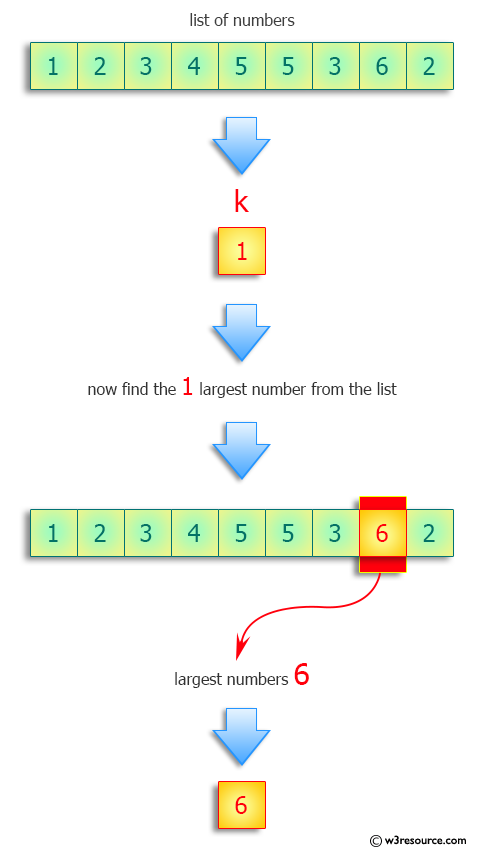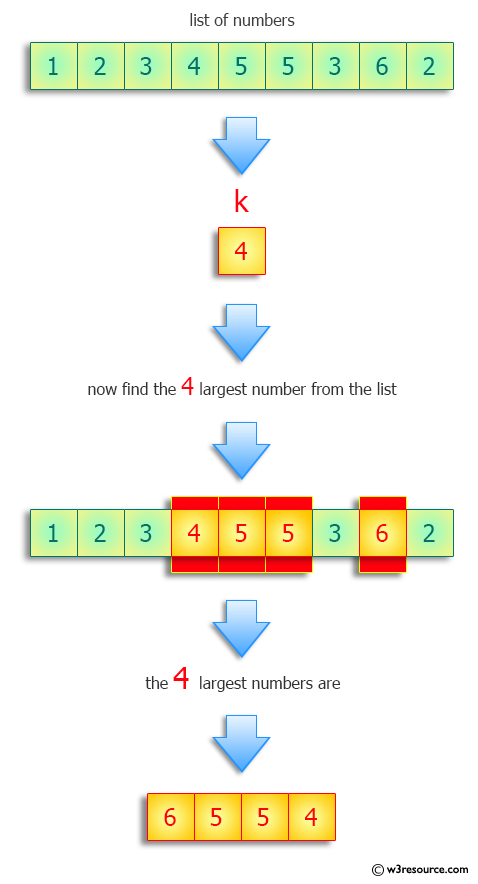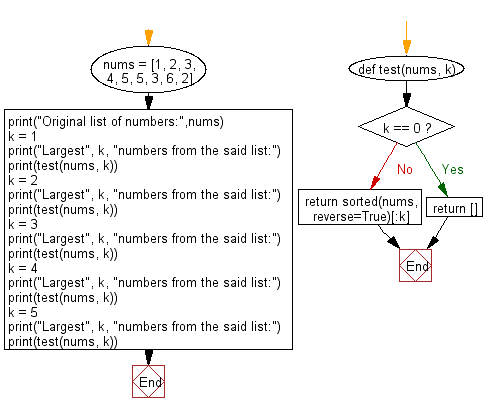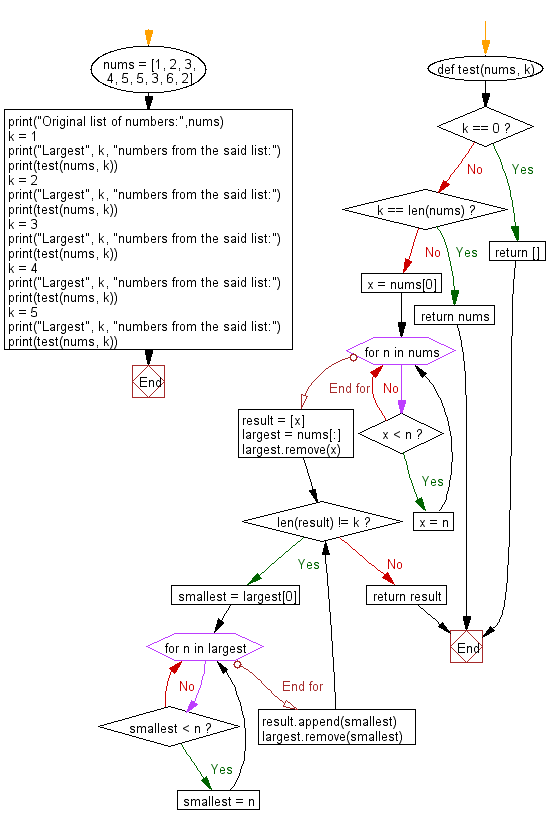﻿ Python: Find the largest k numbers - w3resource# Python: Find the largest k numbers

## Python Programming Puzzles: Exercise-36 with Solution

Write a Python program to find the largest k numbers from a given list of numbers.

```Input:
[1, 2, 3, 4, 5, 5, 3, 6, 2]
Output:

Input:
[1, 2, 3, 4, 5, 5, 3, 6, 2]
Output:
[6, 5]
Input:
[1, 2, 3, 4, 5, 5, 3, 6, 2]
Output:
[6, 5, 5]
Input:
[1, 2, 3, 4, 5, 5, 3, 6, 2]
Output:
[6, 5, 5, 4]
Input:
[1, 2, 3, 4, 5, 5, 3, 6, 2]
Output:
[6, 5, 5, 4, 3]
```

Pictorial Presentation:Sample Solution-1:

Python Code:

`````` #License: https://bit.ly/3oLErEI

def test(nums, k):
if k == 0:
return []
return sorted(nums, reverse=True)[:k]
nums = [1, 2, 3, 4, 5, 5, 3, 6, 2]
print("Original list of numbers:",nums)
k = 1
print("Largest", k, "numbers from the said list:")
print(test(nums, k))
k = 2
print("Largest", k, "numbers from the said list:")
print(test(nums, k))
k = 3
print("Largest", k, "numbers from the said list:")
print(test(nums, k))
k = 4
print("Largest", k, "numbers from the said list:")
print(test(nums, k))
k = 5
print("Largest", k, "numbers from the said list:")
print(test(nums, k))
``````

Sample Output:

```Original list of numbers: [1, 2, 3, 4, 5, 5, 3, 6, 2]
Largest 1 numbers from the said list:

Largest 2 numbers from the said list:
[6, 5]
Largest 3 numbers from the said list:
[6, 5, 5]
Largest 4 numbers from the said list:
[6, 5, 5, 4]
Largest 5 numbers from the said list:
[6, 5, 5, 4, 3]
```

Flowchart:## Visualize Python code execution:

The following tool visualize what the computer is doing step-by-step as it executes the said program:

Sample Solution-2:

Python Code:

``````#License: https://bit.ly/3oLErEI

def test(nums, k):
if k == 0:
return []
elif k == len(nums):
return nums
else:
x = nums
for n in nums:
if x < n:
x = n
result = [x]
largest = nums[:]
largest.remove(x)
while len(result) != k:
smallest = largest
for n in largest:
if smallest < n:
smallest = n
result.append(smallest)
largest.remove(smallest)
return result
nums = [1, 2, 3, 4, 5, 5, 3, 6, 2]
print("Original list of numbers:",nums)
k = 1
print("Largest", k, "numbers from the said list:")
print(test(nums, k))
k = 2
print("Largest", k, "numbers from the said list:")
print(test(nums, k))
k = 3
print("Largest", k, "numbers from the said list:")
print(test(nums, k))
k = 4
print("Largest", k, "numbers from the said list:")
print(test(nums, k))
k = 5
print("Largest", k, "numbers from the said list:")
print(test(nums, k))
``````

Sample Output:

```Original list of numbers: [1, 2, 3, 4, 5, 5, 3, 6, 2]
Largest 1 numbers from the said list:

Largest 2 numbers from the said list:
[6, 5]
Largest 3 numbers from the said list:
[6, 5, 5]
Largest 4 numbers from the said list:
[6, 5, 5, 4]
Largest 5 numbers from the said list:
[6, 5, 5, 4, 3]
```

Flowchart:## Visualize Python code execution:

The following tool visualize what the computer is doing step-by-step as it executes the said program:

Python Code Editor :

Have another way to solve this solution? Contribute your code (and comments) through Disqus.

What is the difficulty level of this exercise?

Test your Programming skills with w3resource's quiz.

﻿

## Python: Tips of the Day

Clamps num within the inclusive range specified by the boundary values x and y:

Example:

```def tips_clamp_num(num,x,y):
return max(min(num, max(x, y)), min(x, y))
print(tips_clamp_num(2, 4, 6))
print(tips_clamp_num(1, -1, -6))
```

Output:

```4
-1
```